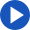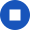## multiply

Regular
Change speakerYou're listening to Joanna
She has an American accent and expects you to use American spelling.

•••••Past

• I multiplied
• you multiplied
• he/she/it multiplied
• we multiplied
• you multiplied
• they multiplied

Present

• I multiply
• you multiply
• he/she/it multiplies
• we multiply
• you multiply
• they multiply

Future

• I will multiply
• you will multiply
• he/she/it will multiply
• we will multiply
• you will multiply
• they will multiply

JoannaYouReset